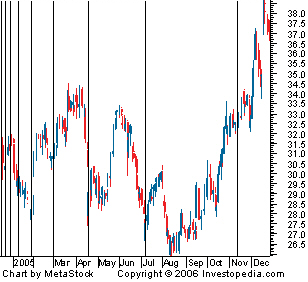# Fibonacci Time Zones

Fibonacci Time Zones
An indicator used by technical traders to identify periods in which the price of an asset will experience a significant amount of movement. This charting technique consists of a series of vertical lines that correspond to the sequence of numbers known as Fibonacci numbers (1, 2, 3, 5, 8, 13, 21, 34, etc.). Once a trader chooses a starting position (most commonly following a major move) on the chart, a vertical line is placed on every subsequent day that corresponds to the position in the Fibonacci number sequence.Fibonacci numbers are a sequence of numbers where each successive number is the sum of the two previous numbers. For reasons unknown, these numbers play an important role in determining relative areas where the prices of financial assets experience large price moves or change direction. The four popular Fibonacci studies are arcs, fans, retracements and time zones.

Investment dictionary. . 2012.

### Look at other dictionaries:

• Fibonacci Numbers/Lines — Leonardo Fibonacci was an Italian mathematician born in the 12th century. He is known to have discovered the Fibonacci numbers, which are a sequence of numbers where each successive number is the sum of the two previous numbers. e.g. 1, 1, 2, 3,… …   Investment dictionary

• Индикаторы цикла — индикаторы, предназначенные для выявления циклических составляющих. Обычно индикаторы цикла хорошо работают только на боковых трендах. К индикаторам цикла относятся Fibonacci time zones, MESA sine wave indicator и др. См. также: Рыночные… …   Финансовый словарь

• 4 (number) — Four redirects here. For other uses, see 4 (disambiguation). 4 −1 0 1 2 3 4 5 6 7 8 9 → List of numbers Integers …   Wikipedia

• William H. Starbuck — Infobox Scientist name = William Starbuck image width = 150px caption = birth date = birth date|1934|9|20|mf=y birth place = Portland, Indiana residence = citizenship = nationality = ethnicity = field = Cognitive Psychologist Organizational… …   Wikipedia